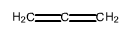🤓 Based on our data, we think this question is relevant for Professor Battin's class at WVU.

# Solution: Predict the approximate bond angles in the following molecule.a. C C-C angleb. H-C-H angle

###### Problem

Predict the approximate bond angles in the following molecule.

a. C C-C angle
b. H-C-H angle

###### Solution

Determine the electron geometry and bond angle using this:

Electron Regions          Electronic Geometry          Bond Angles

2                                      linear                                    180˚

3                                      trigonal planar                      120˚

4                                      tetrahedral                            109.5˚

5                                      trigonal bipyramidal              90˚, 120˚, and 180˚

6                                      octahedral                            90˚ and 180˚View Complete Written Solution